# Microsoft Access Crosstab and Chi-Square with Total Access Statistics

Total Access Statistics extends the basic cross-tabulations available in Microsoft Access. Easily select additional calculation types, summaries, and percentages of row, column and total. The percentages can be created as separate records or as fields in the same record. Additionally, Chi-Square and expected value statistics can be generated to measure the randomness of the distribution.

Total Access Statistics generates crosstabs from your Microsoft Access tables and queries. All results are in MS Access tables that you can use on your forms, reports, and VBA code.

Crosstab Feature Total Access Statistics Microsoft Access
Row SummaryColumn Summary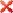Multiple Value Fields% of Column, Row, TotalExpected Cell valueExpected Cell %Chi-Square AnalysisResults in TablesTwo output tables may be created: Cross-tabulation and Chi-Square.

## Introduction of Crosstabs in Microsoft Access

Cross-tabulations show summaries of one field across two fields in a matrix. Crosstabs compare the row field to the column field to display relationships between the values in those two fields quickly in a "spreadsheet" like matrix. Each unique value in the column field is a field in the crosstab result.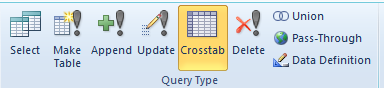Crosstab is a Microsoft Access Query Type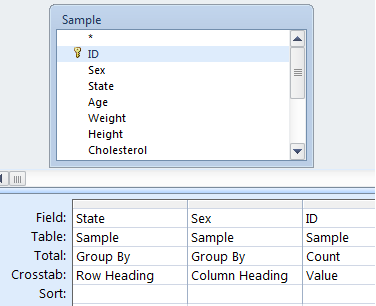Microsoft Access Query Designer for Crosstabs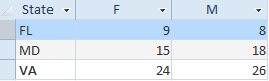Crosstab Results:
Distribution of Counts for State and Gender

## Crosstabs and Chi-Square Features in Total Access Statistics

Total Access Statistics makes it very easy to generate crosstabs and Chi-Square analysis in MS Access:

• Analyze the data from Microsoft Access tables and queries (including linked data)
• An unlimited number of records may be calculated in one pass.
• Optionally specify a weighting field
• Optionally specify a value or range of values to ignore
• Specify one or more ROW fields
• Specify one COLUMN field
• Specify one or more VALUE fields

All the options can be selected interactively through the add-in Wizard interface. VBA developers can run the analysis through a programmatic VBA function call.

## Selecting Fields for Crosstabs

After selecting your data source, field selection is very simple: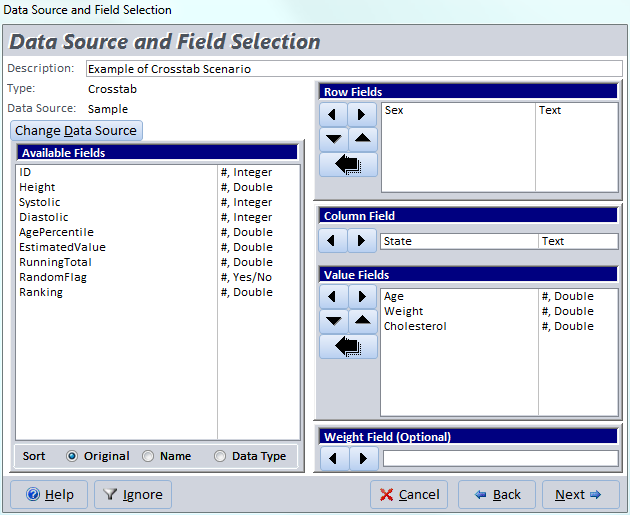Field Selection for Crosstabs: Three value fields are being processed at one time

### Row Fields

Specify at least one row field. The row field(s) becomes the left column(s) of the crosstab. If you choose more than one row field, totals are calculated for the first R-1 row fields (where R is the number of row fields); basically, a separate crosstab for each set of unique values in the first R-1 row fields.

### Column Field

Specify only one column field. Each unique value in the column field becomes a field in the crosstab.

### Value Fields

Specify the fields to be calculated (Sum, Count, etc.) and placed in each of the crosstab's cells. Unlike the Microsoft Access crosstab queries, multiple value fields can be processed at one time.

### Weight Field

As with other types of analysis, you can optionally specify a Weight field. You can also optionally ignore a value or range of values.

After you select your fields and press the [Next] button, the Crosstab options appear.

## Crosstab and Chi-Square Options in Total Access Statistics

Choose among ten Cross-Tabulation types, Percentage, and Chi-Square options: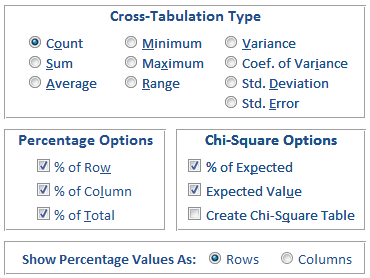### Cross-Tabulation Types

This specifies the type of calculation to perform. If you select a type other than Count, the crosstab generated includes an extra row that shows the Count of each cell. For instance, for Standard Deviation, you would see both the standard deviation for each cell and the number of data points used to calculate the value.

### Percentage Options

You may optionally calculate each cell’s value (based on the Cross-Tabulation Type) relative to its row, column, or total summaries. For percent of column and total, the cell is compared to the corresponding value for its subset of records (unique values in R-1 rows).

### Chi-Square Options

Chi-Square options are available (visible) if the calculation type is Count or Sum. Chi-Square measures the independence of the distribution between the row and column fields.

There are several Chi-Square options. For the main crosstab table, you can add % of Expected values or the Expected value for each cell. Expected values are calculated for each subset. You can also create a separate Chi-Square table that calculates the Chi-Square for each crosstab (subset).

### Show Percentage Value As Option

When you choose to calculate Percentage Options, the values can be shown as rows or columns in the output table. If you choose Rows, the percentage values are shown as additional rows with the value in the DataType field designating what the values are in the row.

If you choose Columns, the percentage values are shown as additional columns, so if you have a column called "Virginia", there would be additional columns like "Virginia%Row", "Virginia%Column", "Virginia%Total", etc. This makes it easy to create reports which show the value and it’s percentages on each row.

Note that because this adds more fields, it can be a problem if your Column field has a lot of unique values (Access has a 255 field limit per table). The default setting is Rows to allow the most unique column values.

The Chi-Square fields (% Expected and Expected) are also displayed in Columns rather than records if you choose the Columns option.

See the output table examples for the result differences.

## Crosstab Output Table

This is a crosstab of the Sample table examining Sex vs. State, with the count of the Age field. This quickly shows how many people fall in each combination of Sex and State: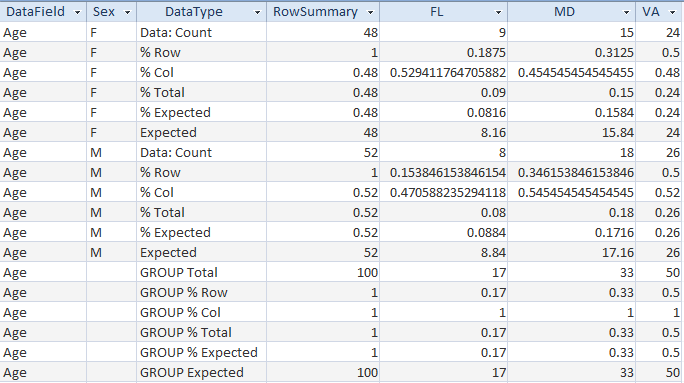Crosstab Output Table with Percentage Values in Rows

### Data Type Field

The [DataType] field shows each record’s calculation including the percent calculations and "GROUP Total" for the crosstab summary:

Data Type Field Value Description
Data: type Cell value where type is the calculation type (Count, Sum, Min, Max, etc.)
% Row Cell's percent of row summary
% Col Cell's percent of subset column
% Total Cell's percent of subset total
% Expected Cell's percent of expected value
Expected Cell's expected value using Chi-Square calculation
GROUP ... Group (subset) summary: Total, %Row, %Col, % Total, etc.

### Row Summary Field

The [RowSummary] field shows the total for the row. This is the summary of the input table data associated with the row and not the values in the crosstab. For instance, the row's average is not the average of the crosstab's cells.

### Column Fields

A field is created for each unique value in the column field. This is case insensitive, i.e. "AAA" is the same as "aaa". If the column field contains a null value, a field named <Null> is created.

### Showing Percentages as Columns in the Crosstab

If the option to Show Percentage Values is set to columns, the output looks like this: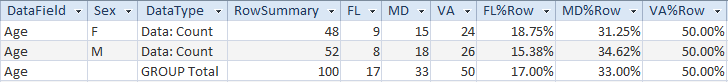Crosstab Output Table with Percentage Values in Columns (truncated with just the first set of fields)

The data is identical to the option with the values in rows, but displayed in columns.

## Chi-Square Output Table

Chi-Square (also known as Pearson's Chi-Square) is a test to determine if the variables in the crosstab row and column fields are independent. The results are placed in a separate table. One record is created for each subset (unique combination of the first R-1 fields, where R is the number of row fields). If there is only one row field, only one record is created per value field.

Chi-Square is only calculated if the Crosstab type is Count or Sum. If a Sum crosstab is selected, all values in the Value field must be positive. Chi-Square analysis of Sum crosstabs is intended for data that contains summed counts.Chi-Square Output Table

### Chi-Square Output Fields

Field Name Description
DataField Field name identifying the value field
Count (N) Number of records (or sum of weightings)
MinExpected Minimum cell's expected value
MaxExpected Maximum cell's expected value
Columns (C) Number of columns with data (some subsets may have no values for a column)
Rows (R) Number of rows with data
DF Degrees of freedom: = (C-1) x (R-1)
Chi-Square (χ2) A sum over all the cells for the difference between the observed and expected values
Prob Probability that the rows and columns are independent based on Chi-Square and DF
Phi-Coefficient Phi-Coefficient statistic
Coeff_of_Contingency Coefficient of Contingency statistic
Cramer's_V Cramer's V statistic

## Interactive Wizard and VBA Programmatic Interfaces

Total Access Statistics lets you interactively generate your calculations through its wizard interface to easily select the data source, fields, and analysis without programming. After you specify your selections, they are automatically saved as a scenario in your database so you can run them again in the future when your data changes.

A VBA statistics function is also available so you can generate these results from code. You can easily run any saved scenario by calling a single procedure in the Total Access Statistics VBA library. The library includes a royalty-free runtime license so you can include it with your Access database and distribute it to non-Total Access Statistics owners.

Call the function from a button's OnClick event or other process, and the results are generated for you. You can even hide the built-in user interface so your users don't even know Total Access Statistics is running. They'll be amazed with your statistical analysis capabilities!

## Additional Details of Calculations Available in Total Access Statistics

Here are some additional resources and details about some of the data analysis you can perform on your Microsoft Access data with Total Access Statistics:

## Total Access Statistics

### Microsoft Access 2016 and 2013 Versions are shipping!

Version 14.1 for
Microsoft Access 2010

Version X.9 for
Microsoft Access 2007 and 2003

Version X.8 for
Microsoft Access 2002, 2000, and 97

### Reviews

"Total Access Statistics performs a wide range of data analysis right inside Access. The product includes a Statistics Wizard to perform parametric, group, non-parametric, record analysis and more. The results are placed in Access tables that you can integrate into your forms and reports."

Alison Balter, Author

More Awards Reviews and References in Scientific Papers

### Statistics Info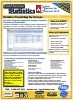PDF Factsheet